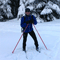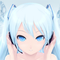# Bug in documentation – form custom validation

Notice: This thread is very old.horakmar
Member | 16
+
0
-

Example in documentation (Forms) doesn't work for me:

``````function divisibilityValidator(\$item, \$arg){
return \$item->value % \$arg === 0;
}

->addRule('divisibilityValidator', 'Number must be divisible by %d.', 8);``````

Issues an error “Validator divisibilityValidator not found”.

Anonymous function works OK:

``````\$divisibilityValidator = function(\$item, \$arg){
return \$item->value % \$arg === 0;
}

->addRule(\$divisibilityValidator, 'Number must be divisible by %d.', 8);``````Machy8
Member | 59
+
0
-

Try this:

``````public function divisibilityValidator(\$item, \$arg)
{
return \$item->value % \$arg === 0;
}

public function createComponentMyForm ()
{
\$form = new Nette\Application\UI\Form;
->addRule(\$this->divisibilityValidator, 'Number must be divisible by %d.', 8);
return \$form;
}``````Aurielle
Member | 1281
+
+3
-

The example from docs doesn't work for you because the code implies a function defined in the global namespace, not a method in the current class, even if the syntax is the same.

A bit of explanation of @Machy8's solution, the `\$this->divisibilityValidator` syntax in `->addRule(\$this->divisibilityValidator, 'Number must be divisible by %d.', 8);` is a bit of a magic, the standard PHP way of specifying a callback would be passing an array, like this:

``````\$form->addText(...)
->addRule([\$this, 'divisibilityValidator'], 'Number must be ...', ...);``````horakmar
Member | 16
+
0
-

OK, thanks for explanation.

Martin